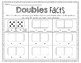Subject
Resource Type
File Type

PDF

(15 MB|54 pages)
Standards
• Product Description
• StandardsNEW
Do your students count on their fingers every time they come across an addition problem? This unit is exactly what you need then. It will teach different strategies to encourage your students to use when adding instead of just counting on.

The following strategies are included:
1. Bridge to 10
2. Using my 10s facts
3. Doubles
4. Near Doubles
5. Counting On
6. Flip Flop Facts
7. Fact Families

Anchor charts, games, practice pages, and addition BINGO are included in the this set.

Use addition bingo to practice all the strategies together.

I promise you will see less fingers being used when adding! This unit would be best for K-2, but could be used for 3rd for your lower students who struggle with mental math.

Let me know if you have any questions!

Ashley
Fluently add and subtract within 20 using mental strategies. By end of Grade 2, know from memory all sums of two one-digit numbers.
Use addition and subtraction within 100 to solve one- and two-step word problems involving situations of adding to, taking from, putting together, taking apart, and comparing, with unknowns in all positions, e.g., by using drawings and equations with a symbol for the unknown number to represent the problem.
Add and subtract within 20, demonstrating fluency for addition and subtraction within 10. Use strategies such as counting on; making ten (e.g., 8 + 6 = 8 + 2 + 4 = 10 + 4 = 14); decomposing a number leading to a ten (e.g., 13 - 4 = 13 - 3 - 1 = 10 - 1 = 9); using the relationship between addition and subtraction (e.g., knowing that 8 + 4 = 12, one knows 12 - 8 = 4); and creating equivalent but easier or known sums (e.g., adding 6 + 7 by creating the known equivalent 6 + 6 + 1 = 12 + 1 = 13).
Relate counting to addition and subtraction (e.g., by counting on 2 to add 2).
Understand subtraction as an unknown-addend problem. For example, subtract 10 – 8 by finding the number that makes 10 when added to 8.
Total Pages
54 pages
N/A
Teaching Duration
N/A
Report this Resource to TpT
Reported resources will be reviewed by our team. Report this resource to let us know if this resource violates TpT’s content guidelines.